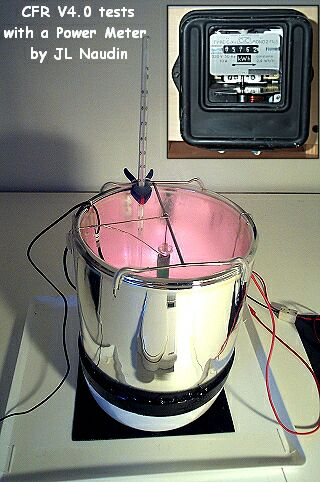Tests of the CFR v4.0 with a Power-Meter
created on May 8, 2003 - JLN Labs - Last update June 16, 2003
Toutes les informations et schémas sont publiés gratuitement ( freeware ) et sont destinés à un usage personnel et non commercial
All informations and diagrams are published freely (freeware) and are intended for a private use and a non commercial use.

The purpose of this test is to measure the electrical energy input of the Cold Fusion Reactor ( CFR ) v4.0 with a new method. Here, in this test, I have used an EDF power meter which is an electromechanical energy-meter ( type C1 X3 CdC Mono 2 fils 220V 50 Hz 10A, 2.4 Wh/tr ). This power meter is connected between the power grid ( 220V AC 50Hz ) and the input of the Variac Transformer of the CFR power supply.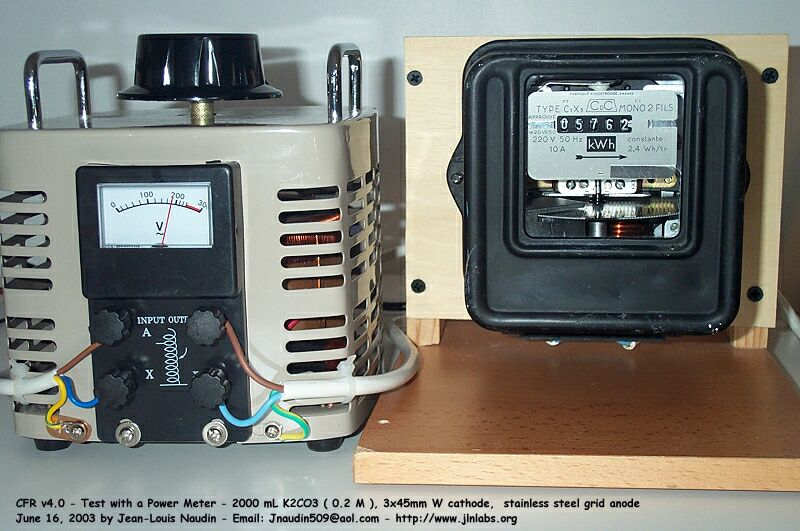In previous tests, I have already used other means such as an electronical Power meter EKM265, an analog DC ammeter, a DC current clamp, a 1 ohm current shunt... In all the cases, the electrical power input measurements were fully coherent and in line with the computed power with the digital oscilloscope Fluke 123. For the temperature measurements, I have also used two different means : a mercury thermometer and a type "K" temp probe ( NiCrNi ) connected on a VC506 digital multimeter ( -20°C to +1200 °C with an accuracy of +/- 3% ).

1 - Experimental test setup :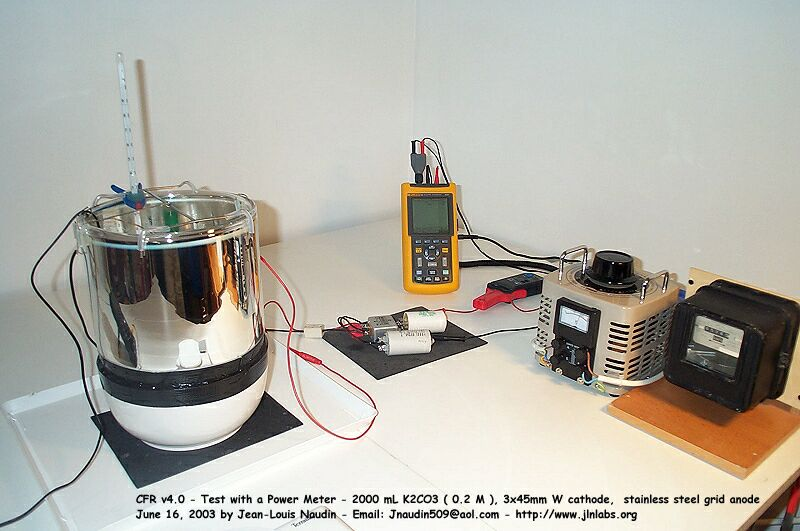The CFR v4.0 is powered with a DC voltage through a bridge rectifier connected through a variable transformer ( Variac ) to the 220V AC power grid line. The voltage input has been measured with a digital oscilloscope Fluke 123 with a Shielded Test Lead STL 120 ( 1:1, 1 Mohms/225 pF ). The current input has been measured with a current clamp CIE Model CA-60A ( Accuracy DC Amps ±1.5%, AC Amps±2% (40Hz-2kHz), AC Amps±4%(2kHz-10kHz), AC Amps±6%(10kHz-20kHz) ). The Power Meter C1 X3 is connected at the input of the variac and to the Power grid. The temperature has been measured with a type "K" temp probe ( NiCrNi ) connected on a VC506 digital multimeter ( -20°C to +1200 °C with an accuracy of +/- 3% ). ( see the photo above ).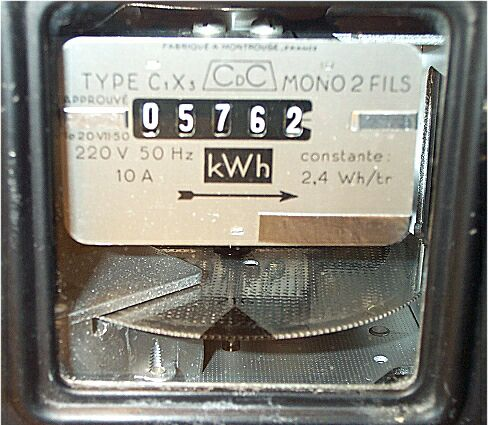Electrical Energy Input measurement method : Initially, the index of wheel of the power meter is placed just in front of the windows. The power meter is rated for 2.4 Wh/tr, this gives 8640 Joules/turn. During this test run, I have counted exactly 21 turns of the wheel, this gives an energy input :

21 turns x 8640 Joules/turn = 181440 Joules

So, it is easy to get an accurate method for measuring the electrical energy required at the input of the CFR.

Test RUN : Test at 220V DC :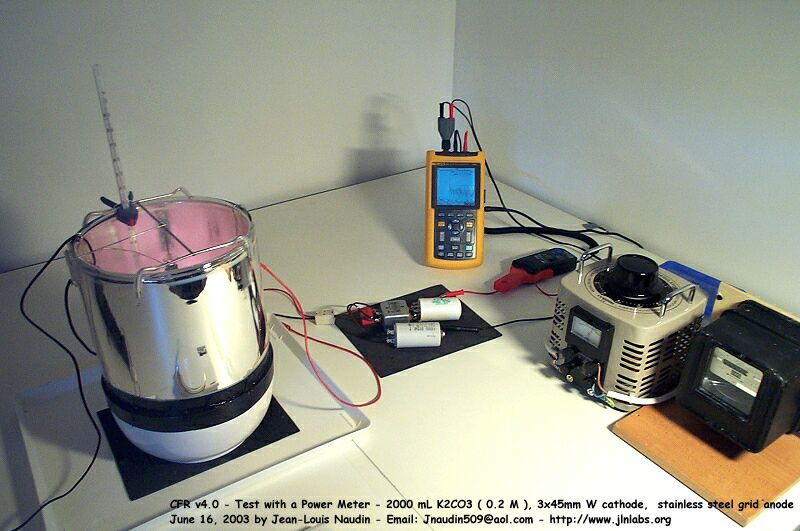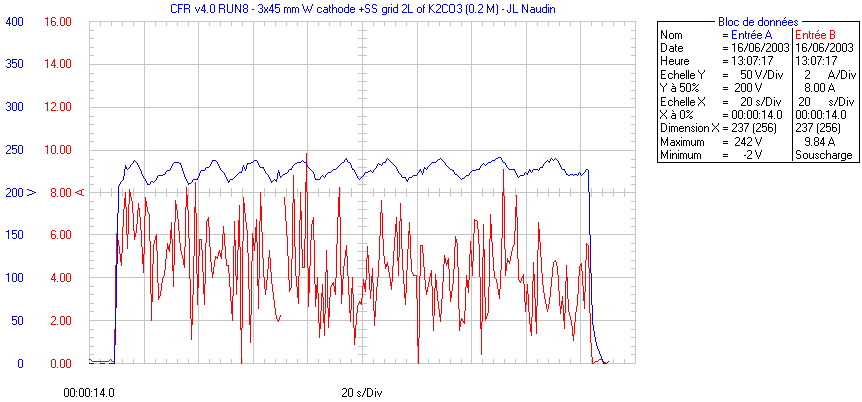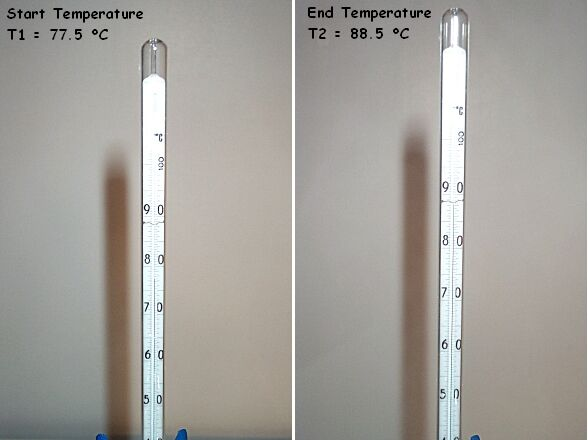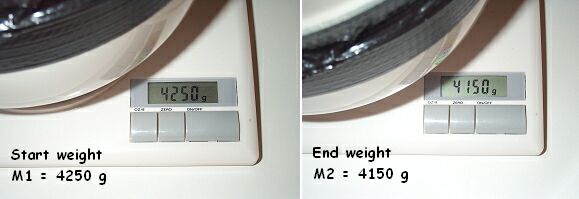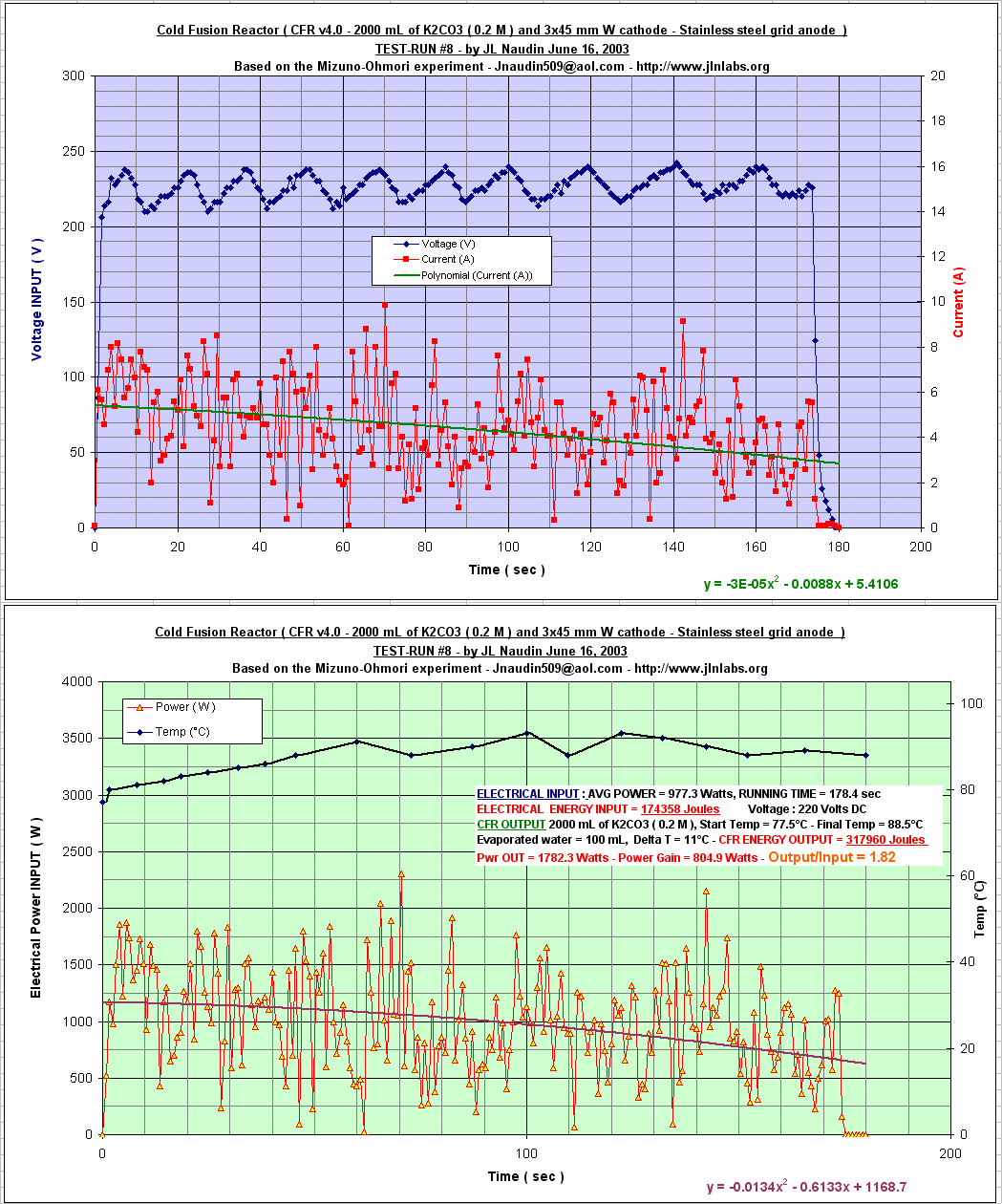Electrolyte solution : 2000 mL of K2CO3 ( 0.2 M ), ELECTRICAL INPUT : AVG POWER = 977.332 Watts, Voltage : 220 Volts RUNNING TIME = 178.4 sec ELECTRICAL ENERGY INPUT at the CFR input = 174358 Joules ELECTRICAL ENERGY INPUT with the Power meter = 181440 Joules CFR OUTPUT : Start Temp = 77.5°C - Final Temp = 88.5°C Evaporated water = 100 mL, Delta T = 11°C CFR ENERGY OUTPUT = 317960 Joules Pwr OUT = 1782.3 Watts - Power Gain = 804.9 Watts COP ( at the CFR input ) = Output/Input = 1.82 COP ( with the Pwr Meter ) = Output/Input = 1.75

Notes from Jean-Louis Naudin : It is interesting to notice that energy input measured with the Fluke 123 is taken at directly at the CFR input and that the energy input measured with the Power meter is taken at the input of the Variac connected to the Power Supply of the CFR. The small difference observed in the electrical energy ( 181440 - 174358 = 7082 Joules or 40 Watts ) can be due to the efficiency of the Variac transformer and the CFR Power Supply ( about 96% ). This new measurements with an EDF electromechanical energy-meter ( type C1 X3 CdC ) confirm again that electrical energy measurements conducted with the Fluke 123 and the current clamp CIE CA-60 are fully coherent and accurate. It is also interesting to notice that during all the CFR v4.0 tests runs, I have always used the same electrolyte K2CO3 solution, I have needed only to add water to complete the level...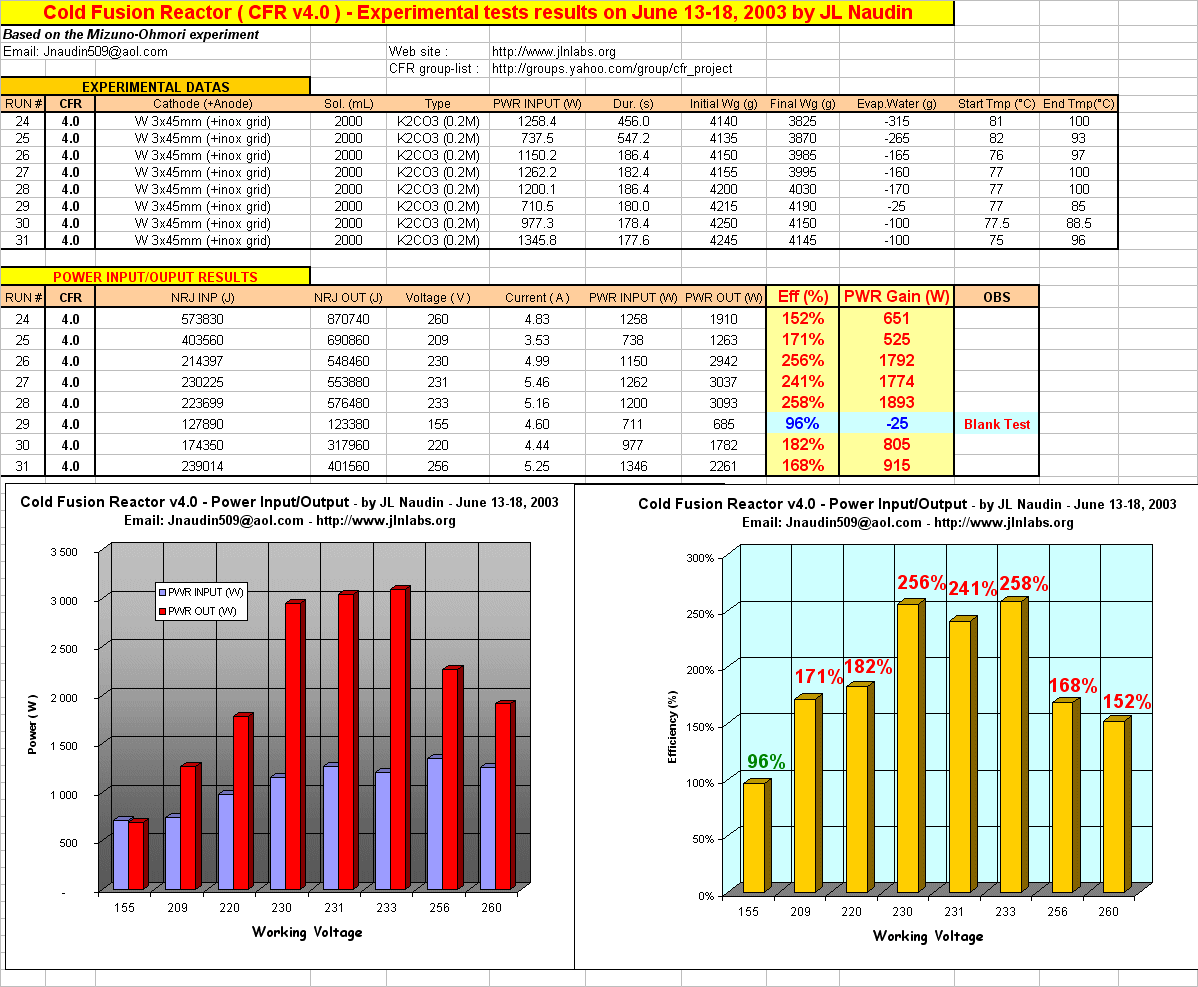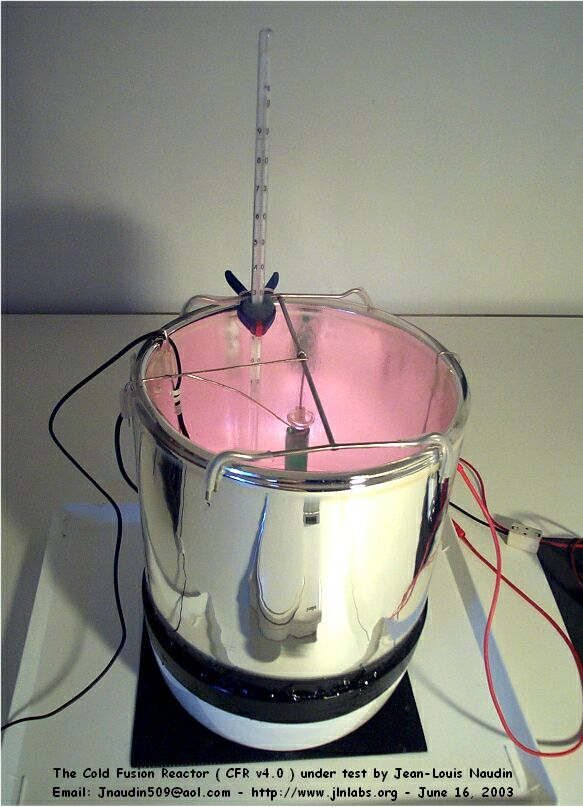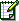Email : JNaudin509@aol.com